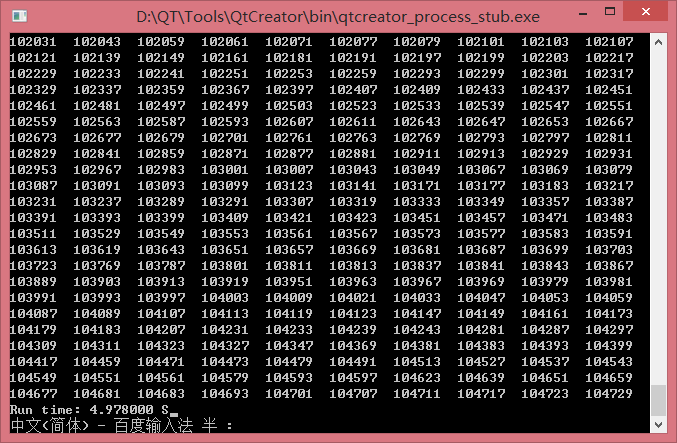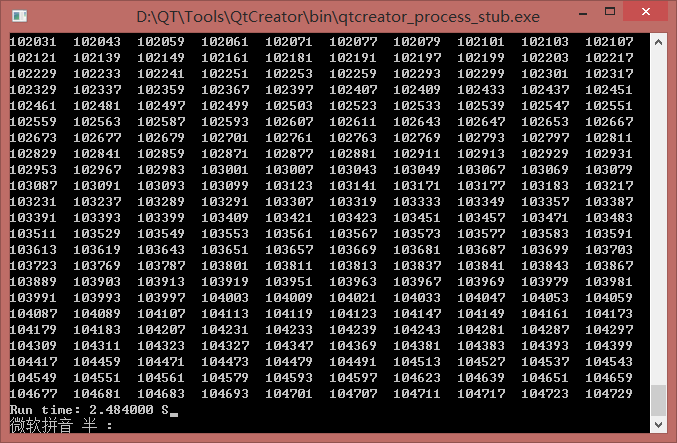## 利用数组求前n个质数 转

``````#include <stdio.h>
#include <time.h>

/** * 利用数组求前n个质数 * 确定一个数m是否为质数，可以用已求出的质数对m * 的整除性来确定 */

//如果不知道质数的特性和想不到优化思路的方法
void getNPrimes_normal();

//优化之后的方法
void getNPrimes_optimize();

int main(void)
{
clock_t start,end;

start = clock(); //开始时，取得开始时间。

//通常的做法的运行时间，输入的n=10000
//getNPrimes_normal();

//优化后的运行时间
getNPrimes_optimize();

end = clock();   //结束时，取得结束时间

printf("Run time: %lf S",(double)(end-start)/CLOCKS_PER_SEC);

return 0;
}

//通常用到的想法
void getNPrimes_normal(){
/** * 用于保存质数的数量 * @brief count */
int count;
printf("Please the count of prime number:\n");

scanf("%d",&count);

//使用数组来保存所求出的质数
int primes[count];

/** * 首先，第一个已知的质数是2， * 则计算应该从3开始 */
primes = 2;
int pc = 1;

int m = 3; //从数字3开始

while(pc < count){

int k = 0;

// 这里只要找不到质数，就会一直在这个循环中
while(k < pc){
if(m % primes[k] == 0){
m += 1;
k = 0;
}else{
k++;
}
}

//找到质数之后，跳出上面的循环
//这个的执行是先执行primes[pc] = m;
//再去执行pc++;
primes[pc++] = m;
m+=1;
}

/** * 对质数进行输出操作 * */
for(pc = 0;pc < count;pc++){
printf("%4d\t",primes[pc]);
}

}

//优化之后的方法
void getNPrimes_optimize(){
/** * 用于保存质数的数量 * @brief count */
int count;
printf("Please the count of prime number:\n");

scanf("%d",&count);

//使用数组来保存所求出的质数
int primes[count];

/** * 首先，第一个已知的质数是2， * 则计算应该从3开始 */
primes = 2;
int pc = 1;

int m =3; //从数字3开始

while(pc < count){

/** * 首先需要解决的是如何判断一个数是一个质数 * 1：除了数字2之外，其他所有的质数都是奇数 * 2：假设某一个数字是k，只要判断k能否被k之前 * 的质数整除就可以了，如果能够整除，则k就是 * 合数，如果不能整除，k就是质数 * * 但是，为了减少算法的复杂度，我们这样设想 * p*q=k * 则肯定p和q中： * p*p <=k的话，q*q >= k * 则，只要求k能否被k的平方根之前的数字整除就可以了。 * * 基于这个思想，我们的实现方式如下： */

int k = 0;

// 这里只要找不到质数，就会一直在这个循环中
while(primes[k] * primes[k] <= m){
if(m % primes[k] == 0){
m += 2; //除了数字2之外，其他所有的质数都是奇数
k = 1; //不用使用数字2去测试
}else{
k++;
}
}

//找到质数之后，跳出上面的循环
//这个的执行是先执行primes[pc] = m;
//再去执行pc++;
primes[pc++] = m;
m+=2;
}

/** * 对质数进行输出操作 * */
for(pc = 0;pc < count;pc++){
printf("%4d\t",primes[pc]);
}
}

``````### 陈洪波

LeetCode：Count Primes - 统计质数数量

1、题目名称 Count Primes（统计质数数量） 2、题目地址 https://leetcode.com/problems/count-primes/ 3、题目内容 英文：Count the number of prime numbers less than a non-negative nu......

2015/09/06
851
0

Xcafe
2016/12/29
4.1K
22

04/30
0
0

P12.2 CommonPrimeDivisors Check whether two numbers have the same prime divisors. P12.2 质因子数 判断两个数是否有相同的因子个数 大于1的自然数中，除了1和它本身以外不再有其他因数的...

AiFan
02/17
0
0
java-生成无重随机序列-10x速~

alvinte
2013/03/01
2.6K
2

100天搞定机器学习|Day55 最大熵模型

1、熵的定义 熵最早是一个物理学概念，由克劳修斯于1854年提出，它是描述事物无序性的参数，跟热力学第二定律的宏观方向性有关：在不加外力的情况下，总是往混乱状态改变。熵增是宇宙的基本定...

32分钟前
5
0

select a.constraint_name, a.table_name, b.constraint_name from user_constraints a, user_constraints b where a.constraint_type = 'R' and b.constraint_type = 'P' and a.r_constrain......

34分钟前
6
0
Web应用安全如何防御或者检查漏洞?

Web应用安全如何防御或者检查漏洞?这是大家一直关心的问题。随着计算机技术的发展，网络漏洞也变得越来越多样化了，你知道吗，每隔9 小时就会发布 1 个严重漏洞，并且有可能会进行远...

42分钟前
7
0
Vim 复制粘帖格式错乱问题的解决办法

43分钟前
7
0
OSM初识（三）OSM Data

yuankaichao
52分钟前
7
0